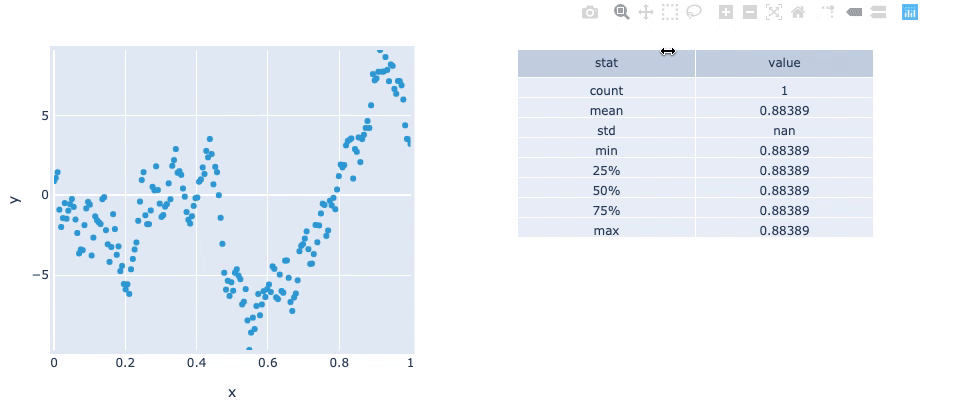# BoundsX#

## Title: BoundsX stream example#

Description: A linked streams example demonstrating how to use BoundsX streams.

Dependencies: Plotly

Backends: Plotly, Bokeh

```import pandas as pd
import numpy as np
import holoviews as hv
from holoviews import streams
hv.extension('plotly')
``````n=200
np.random.seed(20)
xs = np.linspace(0, 1, n)
ys = np.cumsum(np.random.randn(n))
df = pd.DataFrame({'x': xs, 'y': ys})
curve = hv.Scatter(df)

def make_from_boundsx(boundsx):
sub = df.set_index('x').loc[boundsx:boundsx]
return hv.Table(sub.describe().reset_index().values, 'stat', 'value')

dmap = hv.DynamicMap(make_from_boundsx, streams=[streams.BoundsX(source=curve, boundsx=(0,0))])

curve + dmap
```This web page was generated from a Jupyter notebook and not all interactivity will work on this website. Right click to download and run locally for full Python-backed interactivity.